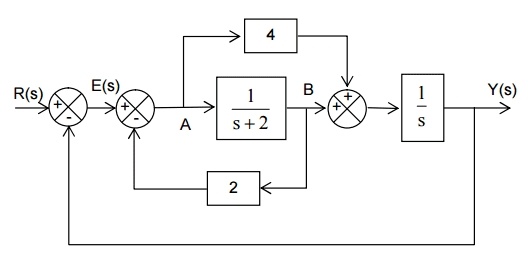# Block Diagram Reduction - Control Systems Engineering

• MechEngJordan

## Homework Statement

Determine the closed loop transfer functions of the systems shown below:## Homework Equations

Standard block diagram algebra.

## The Attempt at a Solution

I've moved the take off point such that the diagram now looks like:I am having difficulty determining G1(s). The solution given is

G1(s) = 4(s + 2) + 1 = 4s + 9

But it is not obvious to me where this is coming from. Taking G1(s) as given, I am able to solve the rest of the question without issue.

Any help appreciated.

The question is a little confusing. The combination of the (s+2) and the gain 4 is clearly 4(s+2). Is that what you mean by G1? If you want to consolidate that loop (second diagram) including the summation block, it would be 4(s+2)+1.

MechEngJordan said:
G1(s) = 4(s + 2) + 1 = 4s + 9
But it is not obvious to me where this is coming from. Taking G1(s) as given, I am able to solve the rest of the question without issue.
.

You have nothing to do than to write down the output of the summing block as a function of the input:
Vout=Vin + Vin*4*(s+2)=Vin(1+4(s+2)).
From this it follows Vout/Vin=4s+9